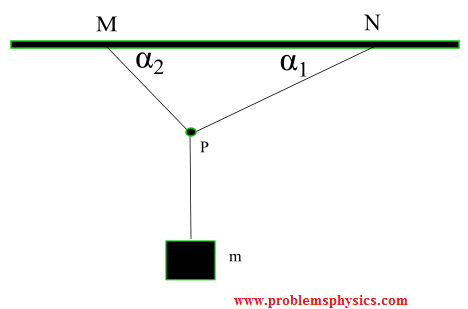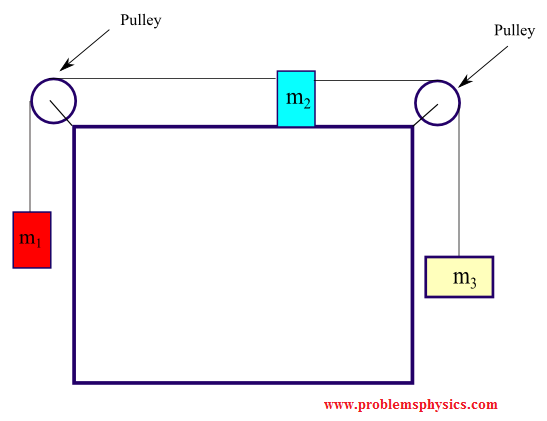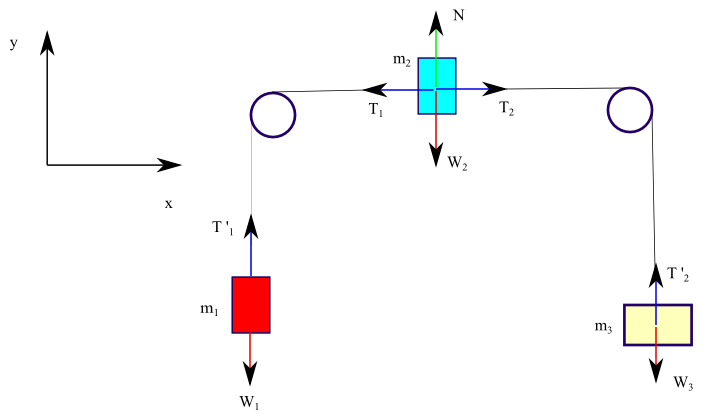# Tension, String, Forces Problems with Solutions

Several problems with solutions and detailed explanations on systems with strings, pulleys and inclined planes are presented. Free body diagrams of forces, forces expressed by their components and Newton's laws are used to solve these problems. Problems involving forces of friction and tension of strings and ropes are also included.

### Problem 1

A block of mass 5 Kg is suspended by a string to a ceiling and is at rest. Find the force Fc exerted by the ceiling on the string. Assume the mass of the string to be negligible.Solution a) The free body diagram below shows the weight W and the tension T1 acting on the block. Tension T2 acting on the ceiling and Fc the reaction to T2.Hence action reaction (Newton's 3 rd law) : |Fc| = |T2| We now consider the forces acting on the block (Free Body Diagram) Since the block is at rest W + T1 = 0 (Newton's second law, vector equation)      W = (0 , -|W|)      T1 = (0, |T1|) Hence : (0 , -|W|) + (0, |T1|) = 0      sum of y coordinates = 0 gives |W| = |T1| T1 and T2 represent the tension of the string and their magnitudes are equal. Hence     |T2| = |T1| T2 and Fc are action and reaction pairs and therefore their magnitudes are equal. Hence     |Fc| = |T2| = |T1| = |W| = m g = 5�10 = 50 N

### Problem 2

In the figure below is shown the system below are shown two blocks linked by a string through a pulley, where the block of mass m1 slides on the frictionless table. We assume that the string is massless and the pulley is massless and frictionless.a) Find the magnitude of the acceleration of the two masses
b) Find the tension in the string
 Solution a)1) free body diagram of block m1 Newton's second law, assuming m1 accelerating from left to right and |a| is the magnitude of the acceleration.      W1 + T1 + N = (m1 |a| , 0) with      W1 = (0 , - |W1|) = (0 , -m1 g)      N = (0 , |N|)      T1 = (|T1| , 0) above equation in components form: (0 , -m1 g) + (0 , |N|) + (|T1| , 0) = (m1 |a| , 0) x components equation      0 + 0 + |T1| = m1 |a| 2) free body diagram of block m2 Newton's second law, assuming m2 accelerating downward and |a| is the magnitude of the acceleration      W2 + T2 = (0 , -m2 |a|)      W2 = (0 , - |W2|) = (0 , - m2 g)      T2 = (0 , |T2|)      (0 , - m2 g) + (0 , |T2|) = (0 , -m2 a) y components equation      - m2 g + |T2| = - m2 |a| Note: |T1| = |T2| tension in the string is the same Combining the equations |T1| = m1 |a|, |T1| = |T2| and -m2 g + |T2| = - m2 |a| found above, we can write      - m2 g + m1 |a| = - m2 |a| Solve for |a|      |a| = m2 g / (m1 + m2) b) Use |T1| = m1 |a| and |a| = m2 g / (m1 + m2) to obtain      |T1| = m1 |a| = m1 m2 g / (m1 + m2)

### Problem 3

In the two blocks of masses m1 and m2 and pulley system below, the pulley is frictionless and massless and the string around the pulley is massless. Find an expression of the acceleration when the block are released from rest.SolutionLet |a| the magnitude of the acceleration of m1 and m2 assuming m1 accelerating upward and m2 accelerating downward. 1) Free body diagram of m1 W1 the weight of m1 T1 the tension of the string at m1 (force exerted by string on m1) a1 (vector) acceleration of m1      W1 + T1 = m1 a1 ( Newton's second law (vector equation) )      W1 = (0 , -|W1|)      T1 = (0 , |T1|)      a1 = (0 , |a|) acceleration assuming m1 accelerating upward.      (0 , -|W1|) + (0 , |T1|) = m1 (0 , |a|) y components equation      -|W1| + |T1| = m1 |a| equation (1) 2) Free body diagram of m2 W2 the weight of m2 T2 the tension of the string at m2 (force exerted by string on m2) a2 (vector) acceleration of m2      W2 + T2 = m2 a2 ( Newton's second law (vector equation))      W2 = (0 , -|W2|)      T2 = (0 , |T2|)      a2 = (0 , - |a|) , assuming m2 accelerating downward.      (0 , -|W2|) + (0 , |T2|) = m2 (0 , -|a|) y components equation      - |W2| + |T2| = - m2 |a| equation (2) Use equation (1) and (2) found above to write      |T1| = m1 |a| + |W1|      |T2| = - m2 |a| + |W2| T1 and T2 represent the tension in the same string and therefore their magnitudes are equal. Using the fact that |T1| = |T2| and the last two equations to write      m1 |a| + |W1| = - m2 |a| + |W2| W1 and W2 are the weights of m1 and m2 and are given by      |W1| = m1 g and |W2| = m2 g Substitute in the above equation to write      m1 |a| + m1 g = - m2 |a| + m2 g Solve for |a| to obtain      |a| = g ( m2 - m1 ) / (m1 + m2)

### Problem 4

Three cords are knotted at point P, with two of these cords fastened to the ceiling making angles α1, α2 and a block of mass m hangs from the third one as shown below.a) Find the magnitude of the tension in each cord in terms of α1, α2 and m so that the system is at rest.
b) Find numerical values to the three tensions found above for α1 = 45� , α2 = 30� and m = 1 Kg.
 Solutiona) 1) Free body diagram of m      W + T '3 = 0      W = (0 , -|W|) , weight      T '3 = (0 , |T '3|) , tension of string y components equation gives      |T '3| = |W| Tension in the same cord      |T3| = |T '3| = |W| 2) Free body diagram of point P At point p (origin of x y system of axes)      T1 + T2 + T3 = 0 (Newton's second law)      T1 = ( |T1| cos α1 , |T1| sin α1)      T2 = ( -|T2| cos α2 , |T2| sin α2)      T3 = (0 , -|T3|) = (0 , -|W|) , (since |T3| = |T '3| = |W| already found above) sum of x components = 0 gives equation      |T1| cos α1 - |T2| cos α2 = 0 (equation 1) sum of y components = 0 gives equation      |T1| sin α1 + |T2| sin α2 - |W| = 0 (equation 2) The above is a system of 2 equations with 2 unknown |T1| and |T2|. Solving the system of equations using Cramer's rules, we obtain      |T1| = |W| cos α2 / ( cos α1 sin α2 + sin α1 cos α2 ) = |W| cos α2 / sin(α1 + α2)      |T2| = |W| cos α1 / ( cos α1 sin α2 + sin α1 cos α2 ) = |W| cos α1 / sin(α1 + α2) b) Numerical application α1 = 45� , α2 = 30� , m = 1 Kg and g = 10 m/s2 |W| = 1�10 = 10 N |T1| = |W| cos α2 / sin(α1 + α2) = 10 cos 30� /sin(45�+30�) = 9.0 N      |T2| = |W| cos α1 / sin(α1 + α2) = 10 cos 45� /sin(45�+30�) = 7.3 N

### Problem 5

The system below includes 3 blocks of masses m1 = 1 Kg, m2 = 2 Kg and m3 = 5 Kg linked by massless and frictionless strings and pulleys.a) Find the magnitude of the acceleration of the 3 blocks.
b) Find the magnitude of the tension of the string between m
1 and m2.
c) Find the magnitude of the tension of the string between m2 and m3.
 Solutiona) We assume that m1 is accelerating upward, m2 from left to right and m3 downward. We apply Newton's second law for each block. |a| is the magnitude of the acceleration of all 3 blocks. 1) Free body diagram of m1      W1 + T '1 = (0 , m1 |a|) (Newton's law)      (0 , -|w1|) + (0 , |T '1|) = (0 , m1 |a|) (above equation using components, equation 1) 2) Free body diagram of m2      W2 + T1 + T2 + N = (m2 |a| , 0)      (0 , - |W2|) + (-|T1| , 0) + (|T2| , 0) + (0 , |N|) = (m2 |a| , 0) (above equation using components, equation 2) 3) Free body diagram of m3      W3 + T '2 = (0 , -m3 |a|)      (0 , - |W3|) + (0 , |T '2|) = (0 , -m3 |a|) (above equation using components, equation 3) equation 1 gives: -|w1| + |T '1| = m1 |a| , y components equal equation 2 gives: -|T1| + |T2| = m2 |a| , x components equal equation 3 gives: - |W3| +|T '2| = -m3 |a| , y components equal We also have      |T '1| = |T1| same tension in the string between m1 and m2      |T '2| = |T2| same tension in the string between m2 and m3 combining all the equations above we obtain      |a| = g (m3 - m1) / (m1 + m2 + m3) Use m1 = 1 Kg, m2 = 2 Kg and m3 = 5 Kg and g = 10 m/s2      |a| = 10 (5 - 1) / (1 + 2 + 5) = 5 m/s2 b)      |T1| = |T '1| = m1 |a| + |w1|      |T1| = |T '1| = 1 � 5 + 1�10 = 15 N c)      |T2| = |T '2| = m2 |a| + |T1| = m2 |a| + m1|a| + |w1|      |T2| = |T '2| = 10 + 15 = 25 N

### Problem 6

In the system below, blocks of masses m1 = 10 Kg and m2 = 30 Kg are linked by a massless string through a frictionless pulley.a) Find the magnitude of the acceleration of the two masses if the coefficient of kinetic friction between the inclined plane and mass m
1 is equal to 0.4.
b) Find the magnitude of the tension in the string.
 Solutiona) 1) Free body diagram of m1      W1 + N + Fk + T = (m1|a| , 0) (second law of Newton vector equation) , where |a| is the mangnitude of the acceleration where      W1 = (W1x, W2x) = (-|W1|sin28� , -|W1|cos28�) , weight of block 1      N = ( 0 , |N|) , normal force      Fk = (-μk |N| , 0) , force of friction opposite motion assuming m1 moving upward      T = (|T| , 0) , tension of string. Rewrite above equation using components      (-|W1|sin28� , -|W1|cos28�) + ( 0 , |N|) + (-μk |N| , 0) + (|T| , 0) = (m1|a| , 0) equality of x components      -|W1|sin28� + 0 - μk |N| + |T| = m1|a| , equation (1) equality of y components      - |W1|cos28� + |N| = 0 which gives |N| = |W1|cos28� , equation (2) 2) Free body diagram of m2      W2 + T ' = (0 , - m2|a|) , where |a| is the magnitude of the acceleration. where      W2 = (0 , -|W2|) , weight of m2      T ' = (0 , |T '|) , tension of string rewrite above equation using components      (0 , -|W2|) + (0 , |T '|) = (0 , - m2|a|) y components equal      - |W2| + |T '| = -m2|a| or |T '| = |W2| - m2|a| , equation (3) We now use the fact that the tension in the string is the same in magnitude, hence      |T '| = |T| = |W2| - m2|a| Substitute |T| by |W2| - m2|a| and |N| by |W1|cos28� in equation (1) to obtain      - |W1|sin28� - μk |W1|cos28� + |W2| - m2|a| = m1|a|      |a| (m1 + m2) = |W2| -|W1|sin28� - μk |W1|cos28�      |a| = g (m2 - m1 sin28� - m1 μk cos28�) / (m1 + m2) Numerical application with m1 = 10 Kg and m2 = 30 Kg and μk = 0.4 , g = 10 m/s^2      |a| = 10 (30 - 10(sin28� + 0.4 cos28�)) / (10 + 30) ≈ 5.4 m/s^2 b)      |T'| = |T| = |W2| - m2|a| = m2 g - m2 |a| = 30(10 - 5.4) ≈ 138 N